Function Repository Resource:

# CoplanarQ

Determine whether a set of points lies in a plane

Contributed by: Wolfram|Alpha Math Team
 ResourceFunction["CoplanarQ"][{list1, list2, …}] returns True if the set of points determined by listi lies in a plane.

## Details and Options

ResourceFunction["CoplanarQ"] accepts numeric and symbolic arguments.

## Examples

### Basic Examples (3)

Any set of three points of the same dimension lie in a plane:

 In:=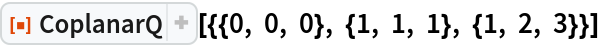Out=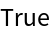In:=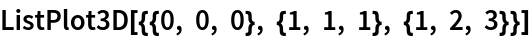Out=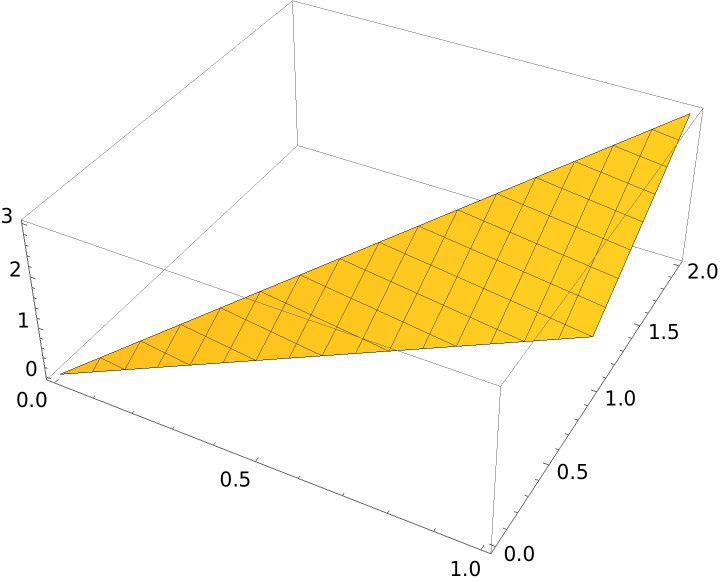Add a fourth point that lies in the same plane:

 In:=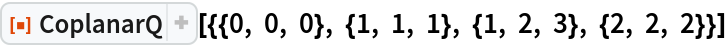Out=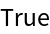In:=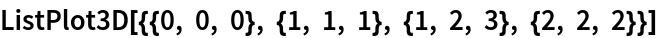Out=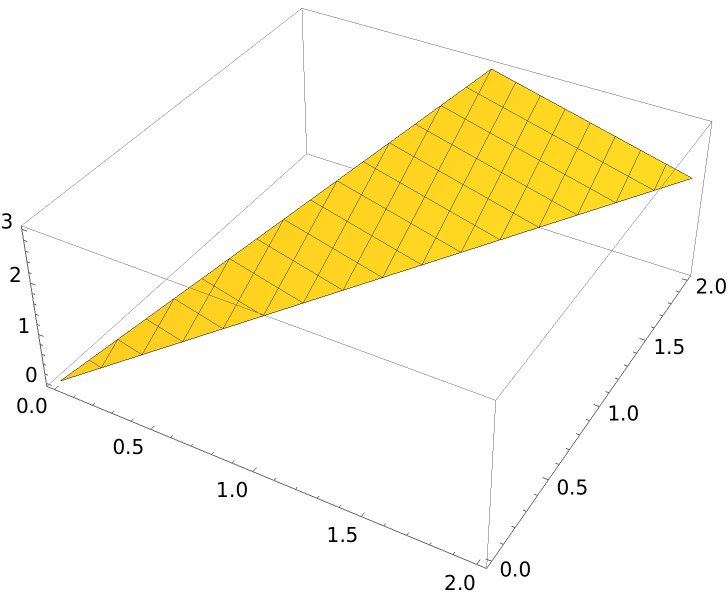A set of four points need not be coplanar:

 In:=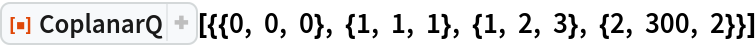Out=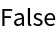In:=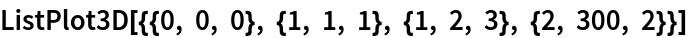Out=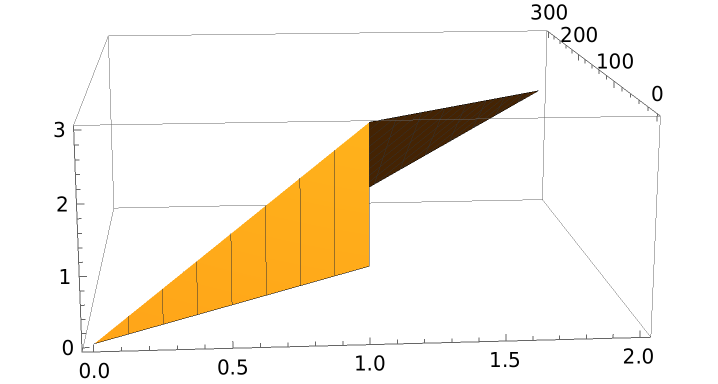### Scope (2)

CoplanarQ works with symbolic arguments:

 In:=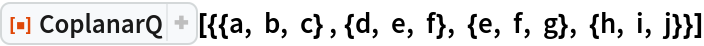Out=In:=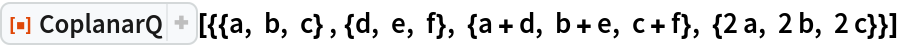Out=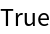CoplanarQ works in higher dimensions as well:

 In:=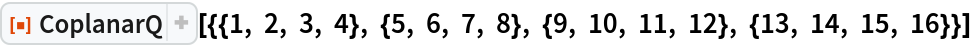Out=In:=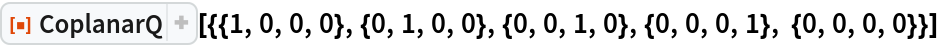Out=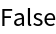## Publisher

Wolfram|Alpha Math Team

## Version History

• 2.0.0 – 23 March 2023
• 1.0.0 – 15 May 2020

## Author Notes

To view the code:

 In:=